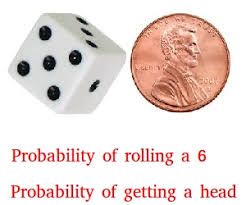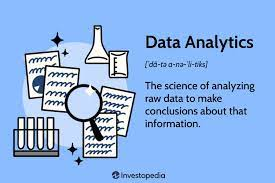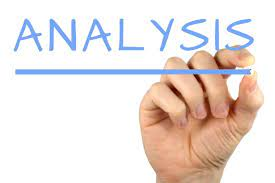I would like to define probability simply as the likelihood of the occurrence of an event.

Probability in statistics means a numerical measure of the likelihood of occurrence.

When we are not sure about the outcome of an event, then we can talk about the probabilities of certain outcomes. The analysis of events using probability is called statistics.

Probability is also known as, likelihood, odd, or chance

BENEFITS OF PROBABILITY

We use or see probability all around us daily. We use probability every day to make decisions about things with an unknown outcome.

Probability is very important in quantifying the unknown. It is used to impose some order into a chaotic world and draw insight from things that are not clear. Therefore, Probability is very useful in making decisions.

SOME TERMINOLOGIES USE IN PROBABILITY

1. Risk: This refers to decision-making situations under which all potential outcomes and their likelihood of occurrences are known to the decision-maker.
2. Uncertainty: This refers to situations under which either the outcomes and/or their probabilities of occurrences are unknown to the decision-maker.
3. Outcome: An outcome is a possible result you can get from doing a trial or experiment. For example, you could get heads when tossing a coin.
4. Sample space: This refers to the set of all possible outcomes or results of an experiment. It is also called sample description space or possibility space.
5. Sample point: Each outcome in a sample space is called a sample point. It can also be called an element or a member of the sample space.
6. Event: An Event is a collection of sample points. It is also a subset of sample space.
7. Compliment of Event: This refers to all the other outcomes (not the ones we want). And together the Event and its Complement make all possible outcomes. Two events are said to be complementary when one event occurs if and only if the other does not. The probabilities of two complementary events add up to 1

There are 3 ways to assign a probability

1. CLASSICAL METHOD: This is a way of assigning probability based on assumptions. Each outcome is assumed to have an equal probability of occurrence. Examples are tossing a coin and rolling a dice. Each sample has an equal likely hood of occurrence.
2. RELATIVE FREQUENCY METHOD: This is a way of assigning probability based on experimentation or historical data.
3. SUBJECTIVE METHOD: This is a way of assigning probability based on personal Intuition or experience, or judgment.

3 TYPES OF DECISION MAKING

1. Decision analysis- This is making a decision with an uncertainty that is using probability

2. Optimization- This refers to decision making with certainty. Looking for the best course of action- The one that maximizes the profit.

3. Sensitivity analysis-This determines how different values of an independent variable affect a particular dependent variable under a given set of assumptions.

PROBABILITY EXAMPLE

What is the probability of rolling a five with one regular six-sided die?

The sample space is {1, 2, 3, 4, 5, 6}. The number of favourable outcomes, or rolling a five, is one.

The number of total outcomes, or all the sides of the die, is six.

So, the probability of rolling a five is 1/6.

In conclusion, a basic knowledge of probability is important as it has several real-life usages. Examples are seen in predicting the weather, sports, insurance and investment analysis.

#MMBA## AWOL & Ajaokuta Steel Mill – April 21 to 23.

in